验证数据集与交叉验证

• 训练数据集和之前的用途一样，是用来训练模型的。
• 验证数据集的作用和之前的测试数据集一样，是用来验证由训练数据集训练出的模型的好坏程度的，或者说是调整超参数使用的数据集。
• 此时的测试数据集和之前的作用就不一样了，这里的测试数据集是当训练出的模型在训练数据集和验证数据集上都表现不错的前提下，最终衡量该模型性能的数据集。测试数据集在整个训练模型的过程中是不参与的。

交叉验证（Cross Validation）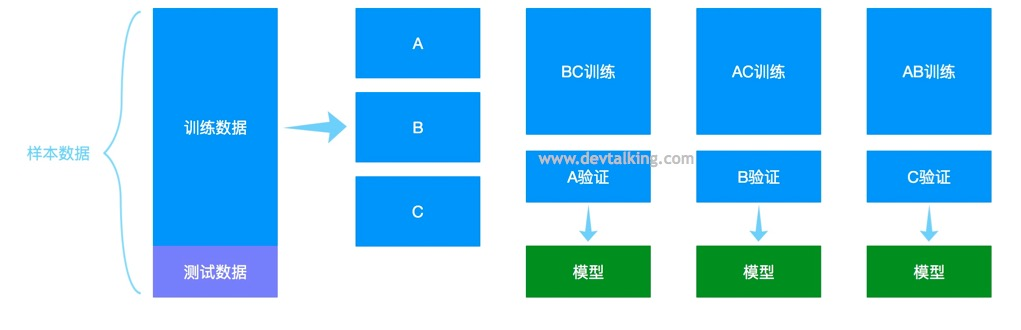偏差（Bias）与方差（Variance）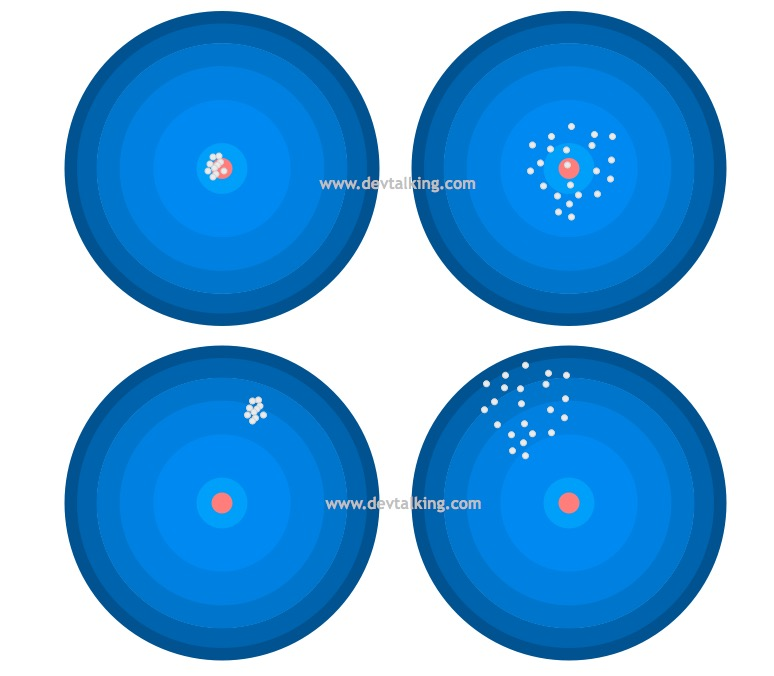• 左上：模型预测的值基本都在目标值上，并且每次预测的都很集中，说明偏差和方差都很小。
• 左下：模型预测的值虽然每次都很集中，但是整体和目标值差的很远，说明偏差很大，方差比较小。
• 右上：模型预测的值基本都围绕着目标值，但是每次预测的值之间差距较大，说明偏差较小，方差比较大。
• 右下：模型预测的值离目标值都很远，并且每次预测的值之间差距也比较打，说明偏差和方差都很大。

• 降低模型复杂度。比如降低多项式回归的degree参数。
• 减少数据维度，降噪。比如使用PCA。
• 增加样本数量。让训练数据足以支撑复杂的模型，从而能计算出合适的参数。
• 使用交叉验证。避免过拟合情况。

模型正则化（Regularization）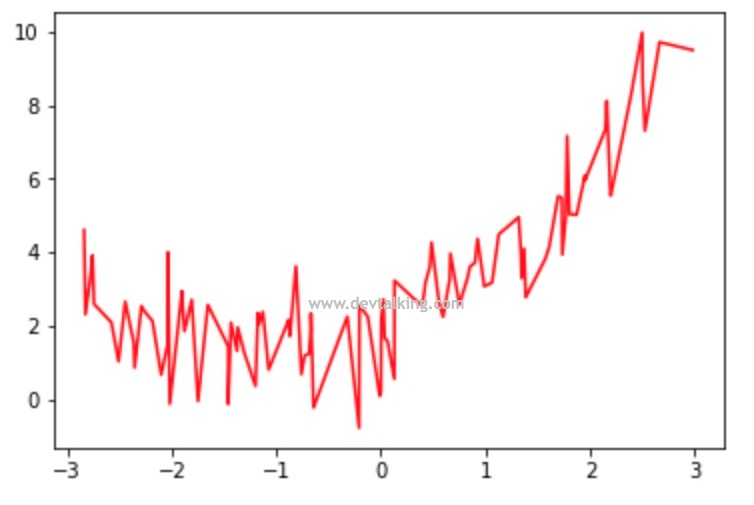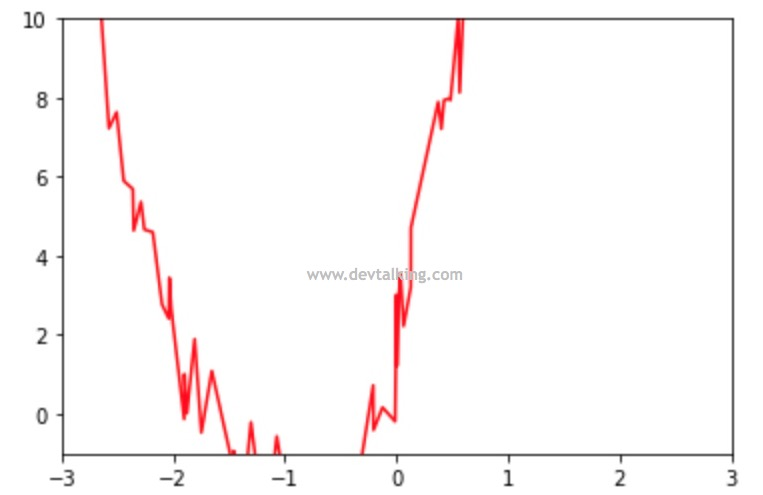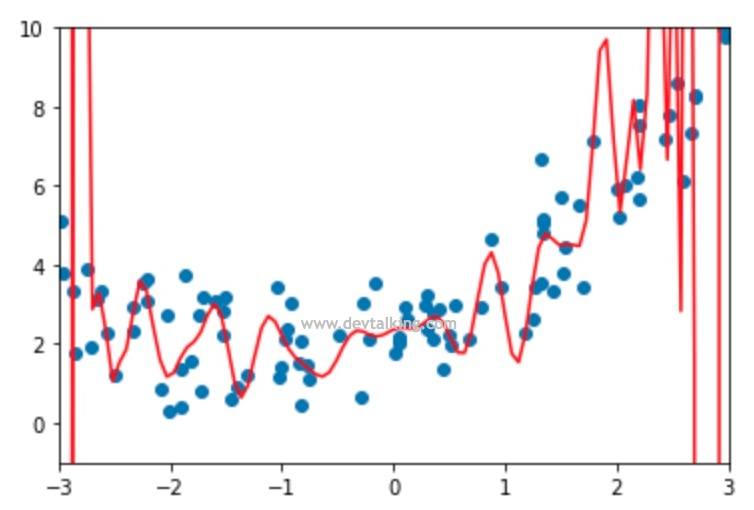$$\sum_{i=1}^m(y^{(i)}- (\theta_0+\theta_1X_1^{(i)}+\theta_2X_2^{(i)}+…+\theta_nX_n^{(i)} ))^2$$

$$L(\theta) = \sum_{i=1}^m(y^{(i)}- (\theta_0+\theta_1X_1^{(i)}+\theta_2X_2^{(i)}+…+\theta_nX_n^{(i)} ))^2 + \alpha \frac 1 2 \sum_{i=1}^n \theta_i^2$$

岭回归（Ridge Regression）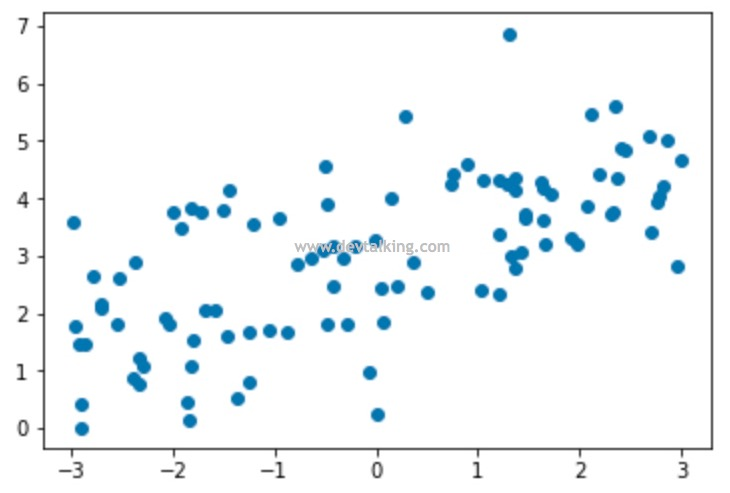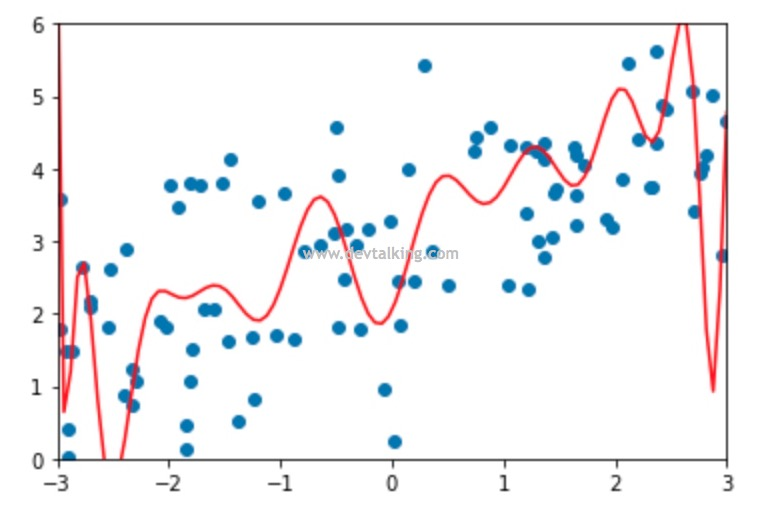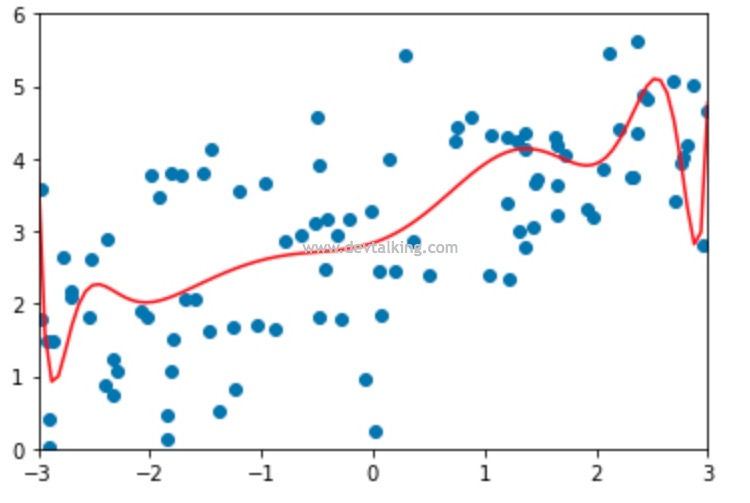LASSO 回归（Least Absolute Shrinkage and Selection Operator Regression）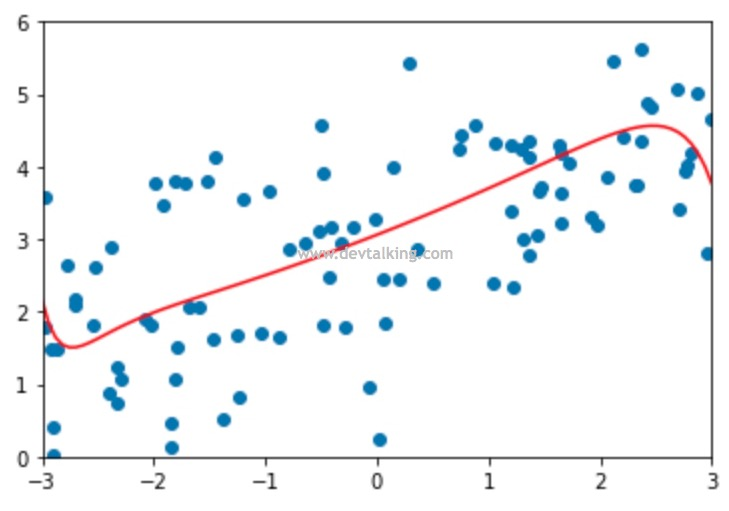岭回归和LASSO回归的数学含义上的区别

$$\nabla = \begin{bmatrix} \theta_1 \\ \theta_2 \\ \theta_3 \\ … \\ \theta_n \end{bmatrix}$$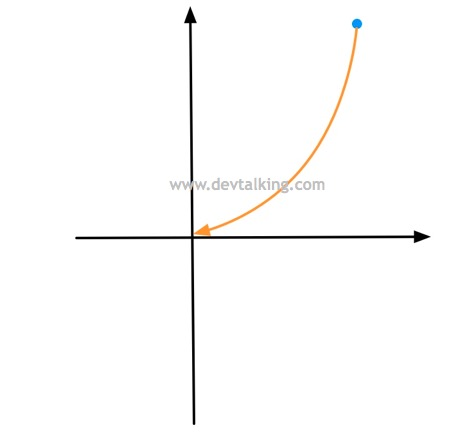$$\nabla = \alpha \begin{bmatrix} sign(\theta_1) \\ sign(\theta_2) \\ sign(\theta_3) \\ … \\ sign(\theta_n) \end{bmatrix}$$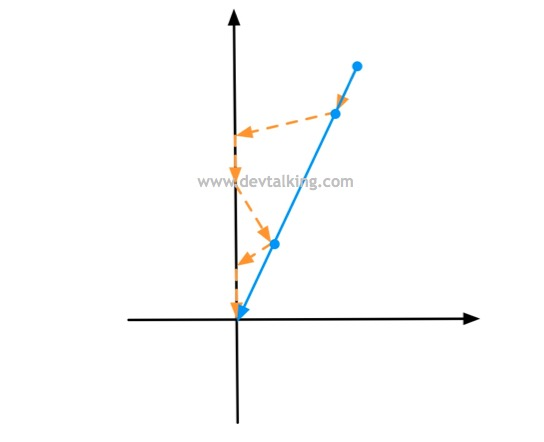LP范数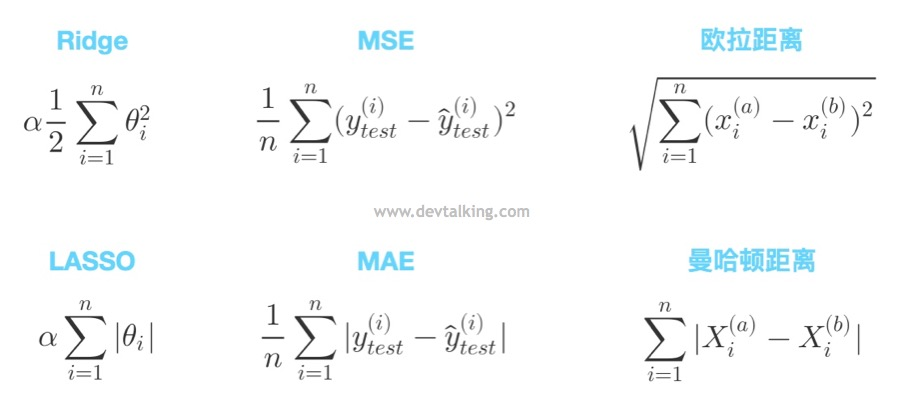$$（\sum_{i=1}^n |X_i^{(a)}-X_i^{(b)}|^p）^\frac 1 p$$

$$||X||_p=(\sum_{i=1}^n|X_i|^p)^{\frac 1 p}$$

弹性网（Elastic Net）

$$L(\theta) = \sum_{i=1}^m(y^{(i)}- (\theta_0+\theta_1X_1^{(i)}+\theta_2X_2^{(i)}+…+\theta_nX_n^{(i)} ))^2 + (1-r)\alpha \frac 1 2 \sum_{i=1}^n \theta_i^2 + r\alpha \sum_{i=1}^n |\theta_i|$$